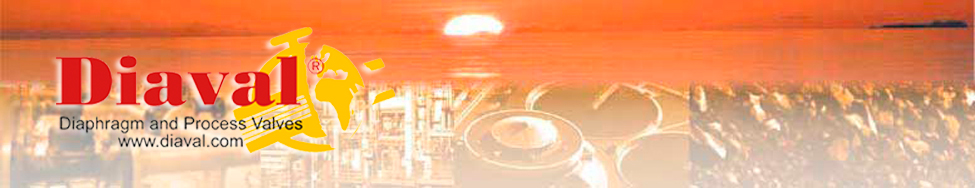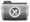Organisations and standardsUNE-EN-ISO StandardsPressure/Temperature relationship chart ANSI B16.34-1988Pressure/Temperature relationship chart DIN 2401Steam ChartFlow rate in pipesSteam ExpansionDirective ATEX 94 9 EC. Scope of application in relation to valvesDirective ATEX - ComevalBasic measures for metal valves in pipes EN 558Steel flanges according to DIN EN1092-1 2001Flange Chart ANSI 150-300Flange chart BS / E-F-J-KFlange chart JIS - 5 KRF - 10 KRF - 16 KRF - 20 KRFEquipment for valves and accessoriesCalculate decimal prefixesCalculate PowerCalculate EnergyCalculate PressureCalculate StrengthCalculate AreaCalculate LengthCalculate TemperatureCalculate Mass (Weight)Calculate Volume